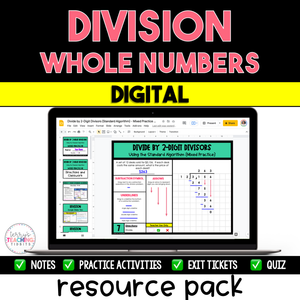# Division of Whole Numbers Resource Pack {Digital}

• \$14.00
Unit price per
Shipping calculated at checkout.

***Scroll down for all bundle options***

This digital resource pack contains everything you need to teach Division of Whole Numbers:

• detailed notes
• student practice
• exit tickets
• quiz

You will successfully help your students learn how to use mental math, the standard algorithm, and partial quotients to divide by 1-digit and 2-digit divisors.

This resource is also included in a 5th Grade Math Year-Long Curriculum Bundle.

Standard:  5.NBT.6

## What You Get

9 Mini-Lessons

Division with Mental Math

• detailed notes with sample problems (Google Slides)
• tutorial video
• 3 practice activities (Google Slides)
• 3 exit tickets (Google Forms)

Division: 1-Digit Divisors {Standard Algorithm}

• 3 mini-lessons
• standard algorithm
• notes with sample problems (Google Slides)
• tutorial video
• zeros in the quotient
• notes with sample problems (Google Slides)
• tutorial video
• remainders
• notes with sample problems (Google Slides)
• tutorial video
• 2 mixed reviews of the 3 types of problems above (Google Slides)
• 6 exit tickets

Division: 1-Digit Divisors {Partial Quotients}

• detailed notes with sample problems (Google Slides)
• tutorial video
• 2 practice activities (Google Slides)
• 4 exit tickets (Google Forms)

Division: 2-Digit Divisors {Standard Algorithm}

• 3 mini-lessons
• standard algorithm
• notes with sample problems (Google Slides)
• tutorial video
• zeros in the quotient
• notes with sample problems (Google Slides)
• tutorial video
• remainders
• notes with sample problems (Google Slides)
• tutorial video
• 2 mixed reviews of the 3 types of problems above (Google Slides)
• 6 exit tickets (Google Forms)

Division: 2-Digit Divisors {Partial Quotients}

• detailed notes with sample problems (Google Slides)
• tutorial video
• 2 practice activities (Google Slides)
• 4 exit tickets (Google Forms)